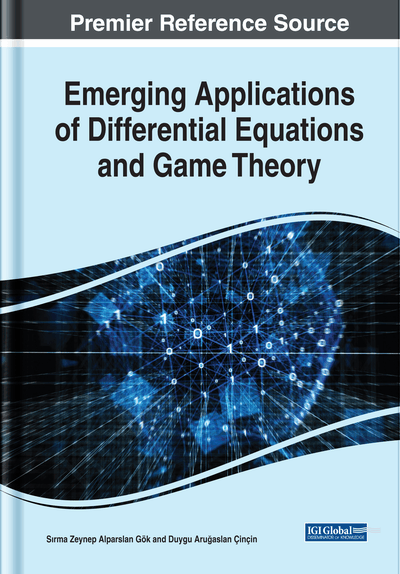# Berezin Number Inequalities of an Invertible Operator and Some Slater Type Inequalities in Reproducing Kernel Hilbert Spaces

Ulaş Yamancı (Süleyman Demirel University, Turkey) and Mehmet Gürdal (Süleyman Demirel University, Turkey)
DOI: 10.4018/978-1-7998-0134-4.ch004
Available
\$37.50
No Current Special Offers

## Abstract

A reproducing kernel Hilbert space (shorty, RKHS) H=H(Ω) on some set Ω is a Hilbert space of complex valued functions on Ω such that for every λ∈Ω the linear functional (evaluation functional) f→f(λ) is bounded on H. If H is RKHS on a set Ω, then, by the classical Riesz representation theorem for every λ∈Ω there is a unique element kH,λ∈H such that f(λ)=〈f,kH,λ〉; for all f∈H. The family {kH,λ:λ∈Ω} is called the reproducing kernel of the space H. The Berezin set and the Berezin number of the operator A was respectively given by Karaev in  as following Ber(A)={A(λ):λ∈Ω} and ber(A):=|A(λ)|. In this chapter, the authors give the Berezin number inequalities for an invertible operator and some other related results are studied. Also, they obtain some inequalities of the slater type for convex functions of selfadjoint operators in reproducing kernel Hilbert spaces and examine related results.
Chapter Preview
Top

## Introduction

Firstly, we will mention about importance of the reproducing kernel as follows (Saitoh & Sawano, 2016; Saitoh, 1988).

The theory of reproducing kernels began with two papers of 1921 (Szego, 1921) and 1922 (Bergman, 1922) which is related with typical reproducing kernels of Szegö and Bergman, and since then the theory has been improved into a large and deep theory in complex analysis by many mathematicians. But, exactly, reproducing kernels appeared previously during the first decade of the twentieth century by S. Zaremba (1907) in his work on boundary value problems for harmonic and biharmonic functions. But he did not improve any further theory for the reproducing property. Furthermore, in fact, we know many concrete reproducing kernels for spaces of polynomials and trigonometric functions from much older days. On the other hand, the general theory of reproducing kernels was established in a complete form by N. Aronszajn (1950) in 1950. Furthermore, L. Schwartz (1964), who is a Fields medalist and founded distribution theory, improved the general theory remarkably in 1964 with a paper of over 140 pages (see Saitoh & Sawano, 2016; Saitoh, 1988).

When linear mappings in the framework of Hilbert spaces are considered, we will encounter in a natural way the notion of reproducing kernels; then the general theory is not restricted to Bergman and Szegö kernels, but the general theory is as important as the concept of Hilbert spaces. It is a main concept and important mathematics. The general theory of reproducing kernels is depending on elementary theorems on Hilbert spaces. The theory of Hilbert spaces is the minimum core of functional analysis. But, when the general theory is combined with linear mappings on Hilbert spaces, it will have many relations in various fields, and its fruitful applications will spread over to differential equations, integral equations, generalizations of the Pythagorean theorem, inverse problems, sampling theory, nonlinear transforms in connection with linear mappings, various operators among Hilbert spaces, and many other broad fields. Furthermore, when we apply the general theory of reproducing kernels to the Tikhonov regularization, it produces approximate solutions for equations on Hilbert spaces which contain bounded linear operators. Looking from the point of view of computer users at numerical solutions, we will see that they are fundamental and have practical applications (see Saitoh & Sawano, 2016; Saitoh, 1988).

Concrete reproducing kernels such as Bergman and Szegö kernels will produce many wide and broad results in complex analysis. They improved some important theory and lead to profound results in complex analysis containing several complex variables. On the other hand, the formal general theory given by Aronszajn also has favorable connections with various fields such as learning theory, support vector machines, stochastic theory, and operator theory on Hilbert spaces (see Saitoh & Sawano, 2016; Saitoh, 1988).

## Complete Chapter List

Search this Book:
Reset